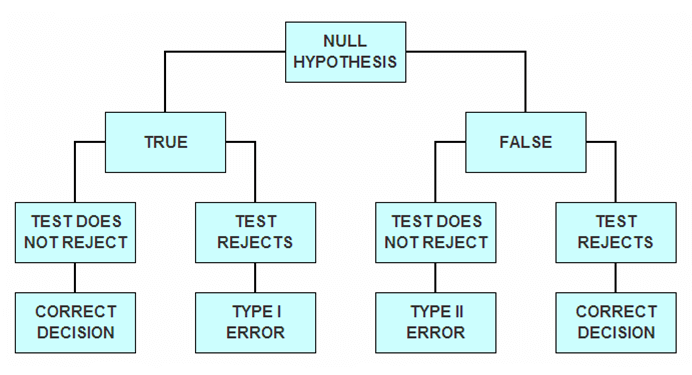StatLect

# Type I error

In a test of hypothesis, a Type I error is committed when the null hypothesis is rejected despite being true.## Synonyms

Type I errors are also called errors of the first kind.

## Explanation

When conducting a test of hypothesis, we decide whether to reject the null hypothesis or not to reject it. Usually the decision is taken based on the value of a test statistic: if the test statistic falls within a pre-specified critical region, then the null is rejected, otherwise it is not rejected. In doing so, we can incur in the following error: reject the null hypothesis when the null hypothesis is indeed true. Errors of this kind are called Type I errors, as opposed to Type II errors, which occur when the null hypothesis is not rejected despite being wrong. This can be summarized in the following diagram.## Probability of Type I errors

As we have already explained, in a test of hypothesis we look at the value taken by a test statistic, and based on this value we decide whether or not tho reject the null hypothesis. The value of the test statistic depends on the data used to perform the test, which is random. Therefore, before observing the data, the test statistic can be seen as a random variable. The probability of committing a Type I error is equal to the probability that this random variable will fall within the critical region when the null hypothesis is true. This probability (or an upper bound to it) is called size of the test, or level of significance of the test.

There is usually a trade-off between the probability of committing Type I errors and the probability of committing Type II errors, that is, a test that is less likely to reject a correct hypothesis is usually also more likely not to reject an incorrect one.

## Example

Suppose you observe a sample of draws from a given distribution, and, based on the sample mean, you reject the null hypothesis that the mean of the distribution is equal to zero. If the true (and unknown) mean of the distribution is indeed equal to zero, then you are committing a Type I error.

A detailed analysis of Type I errors and the probability of committing them when carrying out tests of hypotheses about the mean can be found in the lecture entitled Hypothesis tests about the mean.

## More details

Type I errors are more thoroughly discussed in the lecture entitled Hypothesis testing.